# Painting by Prime Number

Two weeks ago I stumbled upon the concept of prime portraits. In short, prime portraits are pictures in which each color is assigned a number. When we align every pixel, the result should be a prime number. Because I could not find the code used in the article, I decided to recreate these prime portraits in Python – in color! Below is the Jupyter Notebook with the code (also available on Github) I used to generate the Mona Lisa: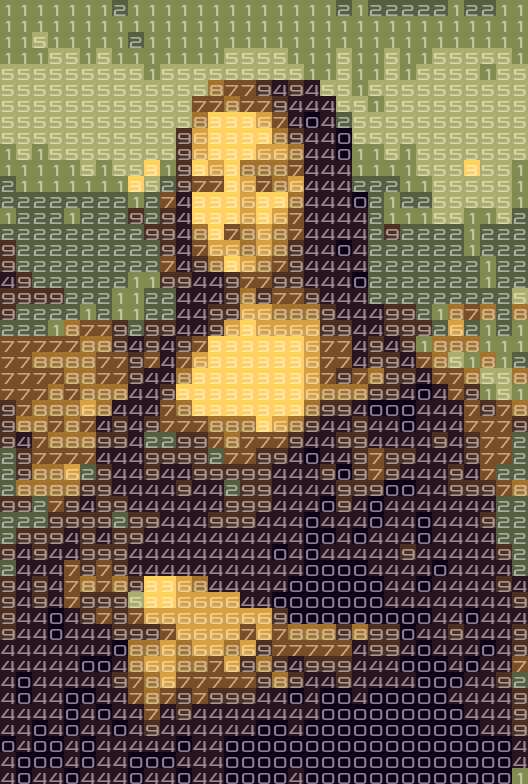## The concept

A prime portrait is a prime number formatted as a matrix with X digits per line. When we select a color for each digit, we can generate an image.

Instead of doing this for many prime numbers and color schemes until you find something that resembles a known image, I have turned the process around. I have taken iconic images, such as the Mona Lisa and Starry Night, and converted them to images with only 10 colors. I assigned a digit to each color. Then I generated many similar images with a little bit of ‘noise’ added. The noise changed the colors in the images slightly, and thus the digits. If the digits in the image formed a prime number, I found a prime portrait!```import matplotlib.pyplot as plt
%matplotlib inline

import numpy as np
import skimage.transform
import skimage.io
from sklearn.cluster import KMeans
import scipy.misc
from PIL import Image, ImageFont, ImageDraw

import numpy as np

from multiprocessing import Pool
import time
import random
import warnings
```

## Testing for primality

When working with extremely long primes, the naive method of testing each divisor will take way too much time. There are a lot of prime numbers and checking your number for each possible divisor takes too long to test a lot of prime portraits.

Instead we take a probabilistic approach to the prime numbers. The Miller Rabin test uses heuristics to determine if a number is (probably) prime or not. I downloaded the following code for the Miller-Rabin primality test from Rosetta code to check for primality:

```def try_composite(a, d, n, s):
# test the base a to see whether it is a witness for the compositeness of n
if pow(a, d, n) == 1:
return False
for i in range(s):
if pow(a, 2**i * d, n) == n-1:
return False
return True # n is definitely composite

def is_probable_prime(n, mrpt_num_trials = 5):
"""
Miller-Rabin primality test.

A return value of False means n is certainly not prime. A return value of
True means n is very likely a prime.

"""
assert n >= 2
# special case 2
if n == 2:
return True
# ensure n is odd
if n % 2 == 0:
return False
# write n-1 as 2**s * d
# repeatedly try to divide n-1 by 2
s = 0
d = n-1
while True:
quotient, remainder = divmod(d, 2)
if remainder == 1:
break
s += 1
d = quotient
assert(2**s * d == n-1)

for i in range(mrpt_num_trials):
a = random.randrange(2, n)
if try_composite(a, d, n, s):
return False

return True # no base tested showed n as composite

assert is_probable_prime(2)
assert is_probable_prime(3)
assert not is_probable_prime(4)
assert  is_probable_prime(5)
assert not is_probable_prime(123456789)
primes_under_1000 = [i for i in range(2, 1000) if is_probable_prime(i)]
assert len(primes_under_1000) == 168
assert primes_under_1000[-10:] == [937, 941, 947, 953, 967, 971, 977, 983, 991, 997]
assert is_probable_prime(6438080068035544392301298549614926991513861075340134\
3291807343952413826484237063006136971539473913409092293733259038472039\
7133335969549256322620979036686633213903952966175107096769180017646161\
851573147596390153)
assert not is_probable_prime(7438080068035544392301298549614926991513861075340134\
3291807343952413826484237063006136971539473913409092293733259038472039\
7133335969549256322620979036686633213903952966175107096769180017646161\
851573147596390153)
```

## Generating the images

The basis of the algorithm is generating images with only 10 possible color values. I did not know what a common approach to this is, so I thought applying a k-means algorithm would work. To do this, I reshaped an input image (so each pixel is a point in 3D space), clustered this, and used the 10 created clusters as color values. Sidenote: when your hammer is machine learning, everything looks like a nail…

Important for the prime generation is that you add a little bit of noise to the original image to create images that look the same, but are slightly different. This means that you created a different number, which is a new chance for us to find a prime number.

```def get_k_means(image):
pointcloud = np.reshape(image, (-1, 3))
kmeans = KMeans(n_clusters=10).fit(pointcloud)
return kmeans

def create_numbered_image(image, kmeans):
"""
Turns an RGB image into an image with the labels of clusters of the supplied kmeans classifier.
"""
# apply noise
stdev = np.std(image)
random_noise = np.random.random_sample(size=image.shape)*(stdev/3)
image = image + random_noise

orig_shape = image.shape
image = np.reshape(image, (-1, 3))

numbered_image = kmeans.predict(image)
numbered_image = np.reshape(numbered_image, orig_shape[:2])

# make sure the end is uneven
if numbered_image[-1,-1]%2==0:
numbered_image[-1,-1] += 1

return numbered_image

def numbered_image_to_normal_image(numbered_image, kmeans):
"""
Turns an image with only values between 0 and 9 into a colored image by using the cluster centers
of the supplied kmeans classifier.
"""
shape = (numbered_image.shape, numbered_image.shape, 3)
image = np.zeros(shape)
for label, color in zip(range(10), kmeans.cluster_centers_):
image[numbered_image==label] = color
return image
```

### Numerised image examples

Here I create a few examples of small numerised images of the Mona Lisa. If you look closely you can see each image is slightly different, but you can still recognise the original picture. This is crucial for us, as this allows us to generate multiple numerised images that we can test for primality.

In :
```def load_and_resize_image(filename, resize_factor=18):
image = image.astype(np.float64)
image = image / 255.

oldshape = image.shape
with warnings.catch_warnings():
warnings.simplefilter("ignore")
resized_image = skimage.transform.resize(image, (oldshape//resize_factor, oldshape//resize_factor))

return resized_image

kmeans = get_k_means(resized_image)
N_IMAGES = 3

f, axarr = plt.subplots(1,N_IMAGES)

for index in range(N_IMAGES):
n_image = create_numbered_image(resized_image, kmeans)
normal_image = numbered_image_to_normal_image(n_image, kmeans)
axarr[index].imshow(normal_image)
```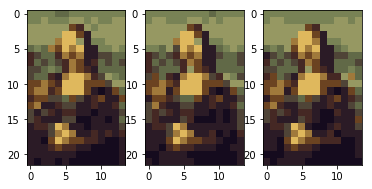## Helper functions

I had to create some helper functions to return strings, integers, and load and show images.

```def image_to_number(numbered_image):
to_be_number = numbered_image.reshape(-1)
as_string = ''.join([str(int(x)) for x in to_be_number])
as_int = int(as_string)
return as_int, as_string

def show_and_save_image(image, n_image, filename, fontsize=16):
oldshape = image.shape
resized_image= scipy.misc.imresize(image,(oldshape*fontsize, oldshape*fontsize), interp='nearest')
img = Image.fromarray(resized_image).convert("RGBA")
txt = Image.new('RGBA', img.size, (255,255,255,0))

draw = ImageDraw.Draw(txt)
font = ImageFont.truetype("pirulen rg.ttf", fontsize)
for y_i, totype in enumerate(n_image):
for x_i, letter in enumerate(totype):
xpos = x_i * fontsize + 1
ypos = y_i * fontsize
if letter == 1:
xpos += 4
draw.text((xpos, ypos),str(letter),(255,255,255, 128),font=font)
img = Image.alpha_composite(img, txt)

img.save(filename)
plt.figure(figsize=(20,20))
plt.imshow(img)
plt.show()

def result_filename(filename):
return filename.split('.') + "-prime.png"
```

To verify if something is a good prime portrait I made the following helper functions:

```def is_good_prime_portrait(n_image):
integer, string = image_to_number(n_image)
if is_probable_prime(integer):
return integer, string, n_image
else:
return None

def print_result(string, n_image):
print(string)
print("-"*100)
for line in n_image:
print(''.join([str(x) for x in line]))
```

At first I created a single-threaded solution, which worked. However, my laptop has multiple cores and could work on multiple numbers at the same time, so I rewrote my initial approach in a multi-threaded fashion:

```def print_result(string, n_image):
print("Found a result: " + "-"*100)
print(string)
print("Represented as portrait:" + "-"*100)
for line in n_image:
print(''.join([str(x) for x in line]))

image_generator = (create_numbered_image(resized_image, kmeans) for _ in range(1000000))
start = time.time()
results = pool.imap_unordered(is_good_prime_portrait, image_generator)
total_results = 0

for result in results:
total_results += 1

# Possibly log time spend searching this prime number
if log_process and total_results%30==0:
elapsed = time.time()
elapsed = elapsed - start
print("Seconds spent in (function name) is {} time per result: {}".format(str(elapsed), str(elapsed/total_results)))

if result != None:
# Found a prime number, print it and save it!
integer, string, n_image = result
print_result(string, n_image)
normal_image = numbered_image_to_normal_image(n_image, kmeans)
plt.imshow(normal_image)
plt.show()
show_and_save_image(normal_image, n_image, result_filename(filename))
break

print("Working with size " + str(resized_image.shape))
kmeans = get_k_means(resized_image)
```

## Generating prime portraits

Now that we defined all functionality, it’s finally time to generate the prime portraits! Play around with it and try to feed your own images. Note that you can pass the resize_factor variable to the function ‘search prime portrait’. The bigger the resize_factor, the faster you can find a prime portrait. See below for more information on the exponential time it takes to generate larger prime portraits.

```folder = 'input_pictures/'

filenames = [
folder + 'monalisa.jpg',
#folder + 'sunflowers.jpg',
#folder + 'starrynight.jpg'
]
filename = filenames

for filename in filenames:
```
```Working with size (19, 13, 3)
Found a result: ----------------------------------------------------------------------------------------------------
6666666666662666626266262222222331222222222395146222066685731062280001758180008000853810000006688771802033388995386733331599578182871883701148387881881131880888181181188883118144141011855514411181111757388411111333881144814118814444114111444444441
Represented as portrait:----------------------------------------------------------------------------------------------------
6666666666662
6666262662622
2222233122222
2222395146222
0666857310622
8000175818000
8000853810000
0066887718020
3338899538673
3331599578182
8718837011483
8788188113188
0888181181188
8831181441410
1185551441118
1111757388411
1113338811448
1411881444411
4111444444441
```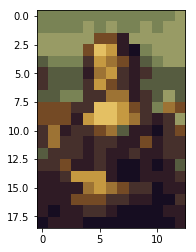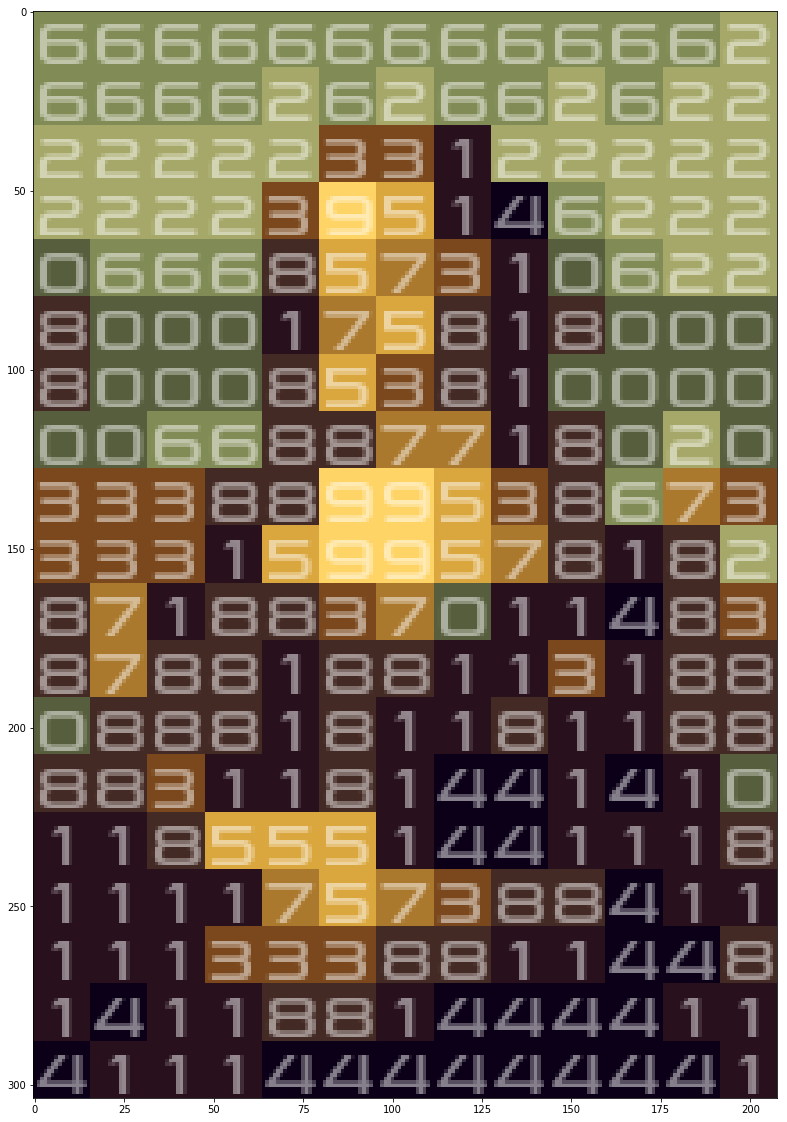## How does this scale?

Apparently the largest known prime number is 22338618 digits long. This would allow us to create a 4726 * 4726 prime portrait, which would be a prime portrait in 4K. I already enjoyed the big images of ‘Starry Night’, and I would absolutely love to have a 4K prime portrait of this painting.

However: I noticed that finding prime numbers does not really scale. The length of the prime number grows quadratically with the resolution of your images, which causes big problems. Although the probability that you find a prime number only decreases linearly with the length of the prime number, it decreases quadratically when you get larger image resolutions. I plotted it in this image to show the effect up to 300×300:

```def trials_expected_img(w,h):
return 2.3*w*h

needed = [trials_expected_img(x,x) for x in range(1,300)]
plt.plot(needed)
```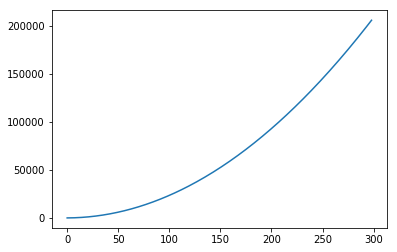But what’s even worse is that computing time grows quadratically with the length of the prime number. I also plotted this for my laptop up to an image resolution of 30×30:

```def time_expected_img(w,h):
order = w*h
start = time.time()
for _ in range(100):
posprim = random.randrange(10**(order-1), 10**order)
a = is_probable_prime(posprim)
end = time.time()
return end - start

test_range = range(2,30,4)
time_needed = [time_expected_img(x,x) for x in test_range]
plt.plot(test_range, time_needed)
plt.title("Seconds expected to calculate if an XxY image is a prime portrait ")
plt.xlabel("Width image")
plt.ylabel("Expected seconds")
```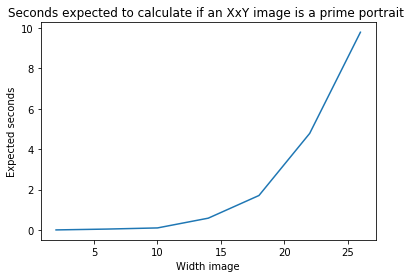Combining both linear curves you can easily estimate the expected time you need to find a portrait of a certain size:

```total_needed = [trials_expected_img(x,x)*time_expected_img(x,x) for x in test_range]
plt.plot(test_range, total_needed)
plt.title("Seconds expected to find an XxY prime portrait ")
plt.xlabel("Width image")
plt.ylabel("Expected seconds")
```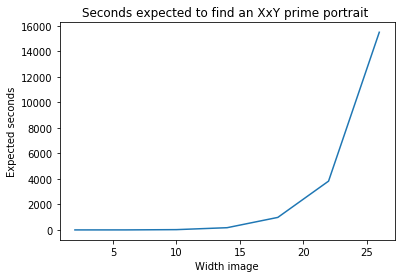If you can find a more efficient Python implementation of a probabilistic prime checker, or implement it in a language more suitable to high demand computation, you can lower the time you need to generate these portraits. Each additional computer core also reduces this time a bit, but for now we can not expect 4K prime portraits anytime soon.

## Conclusion and artworks

The last couple of weeks I really enjoyed letting my computer find prime portraits. Here are some of the artworks my computer found: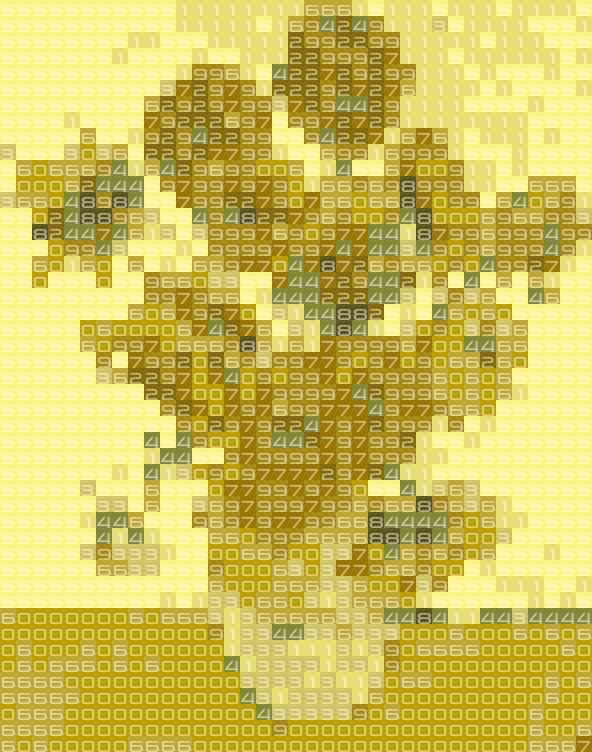Sunflowers by Van Gogh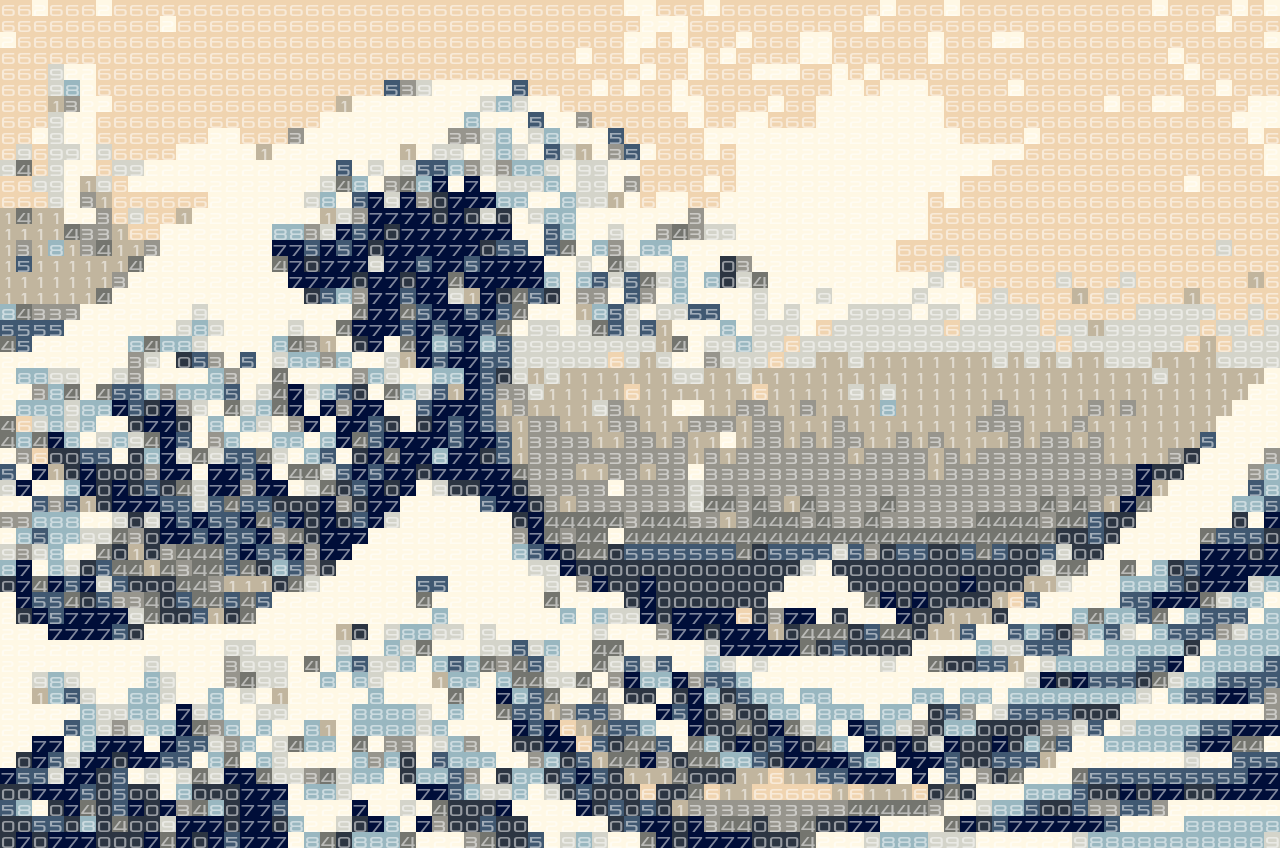Great wave by Hokusai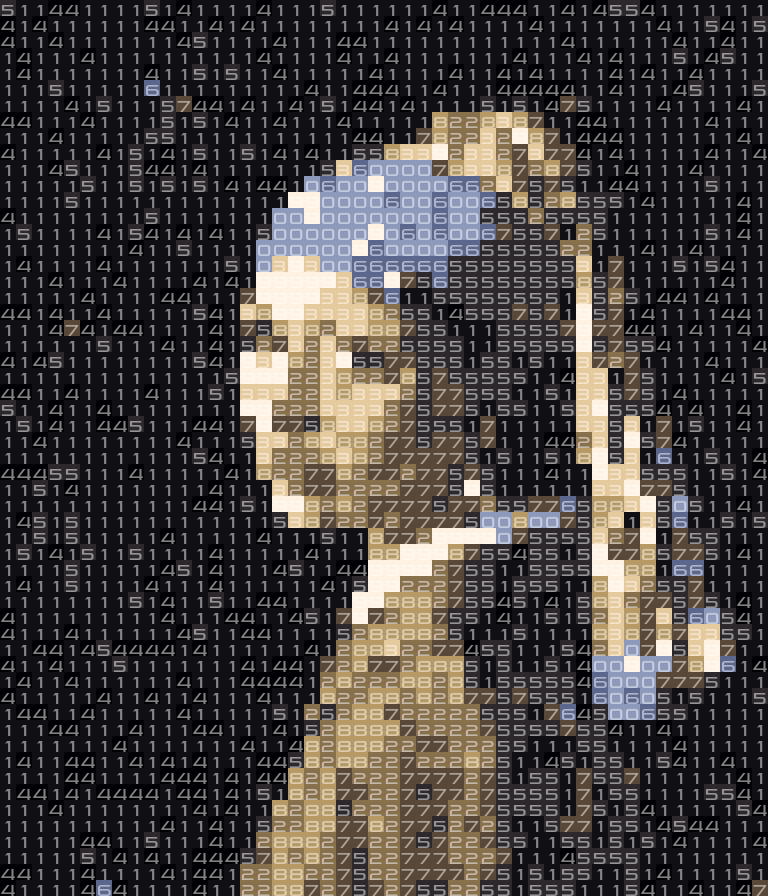Girl with a pearl earring by Vermeer

## Celebrity artworks

Although pictures of existing art often turn our pretty well, it is quite difficult to create good looking prime portraits of people. There is often not enough contrast in the resulting images, which uses only ten colors. I decided to try some portraits for people I like to follow online. Images that turned our well are:

#### Ionica Smeets

Writes interesting articles in a Dutch newspaper every Saturday, and got featured a while ago in the Dutch comic called “Donald Duck”:Makes interesting videos about mathematical concepts on the Numberphile Youtube channel. Also: has a cute dog 😉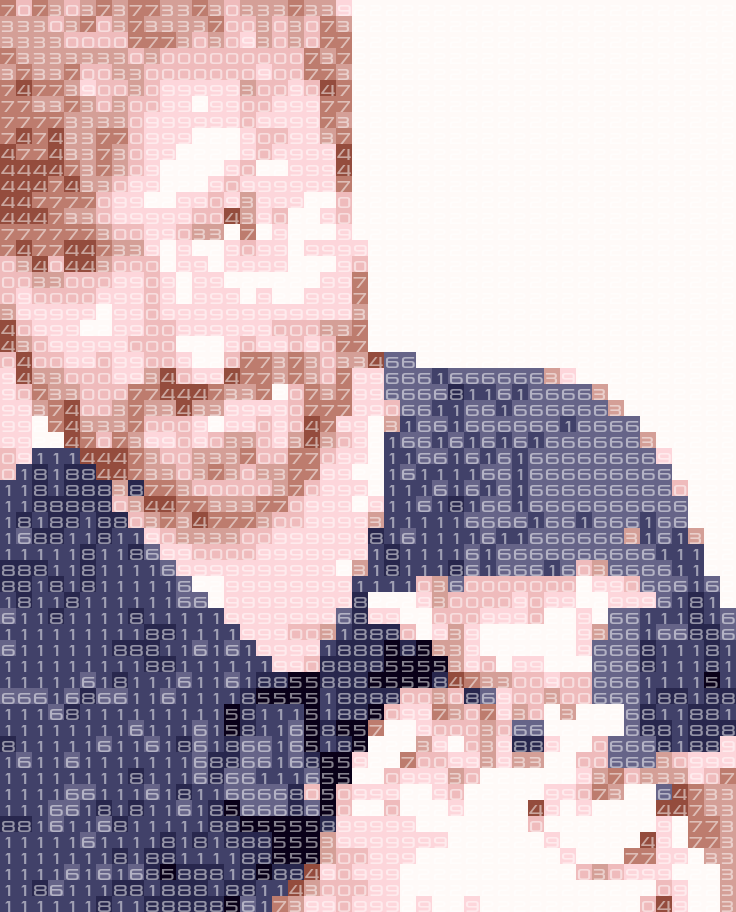#### Roland Meertens

And myself.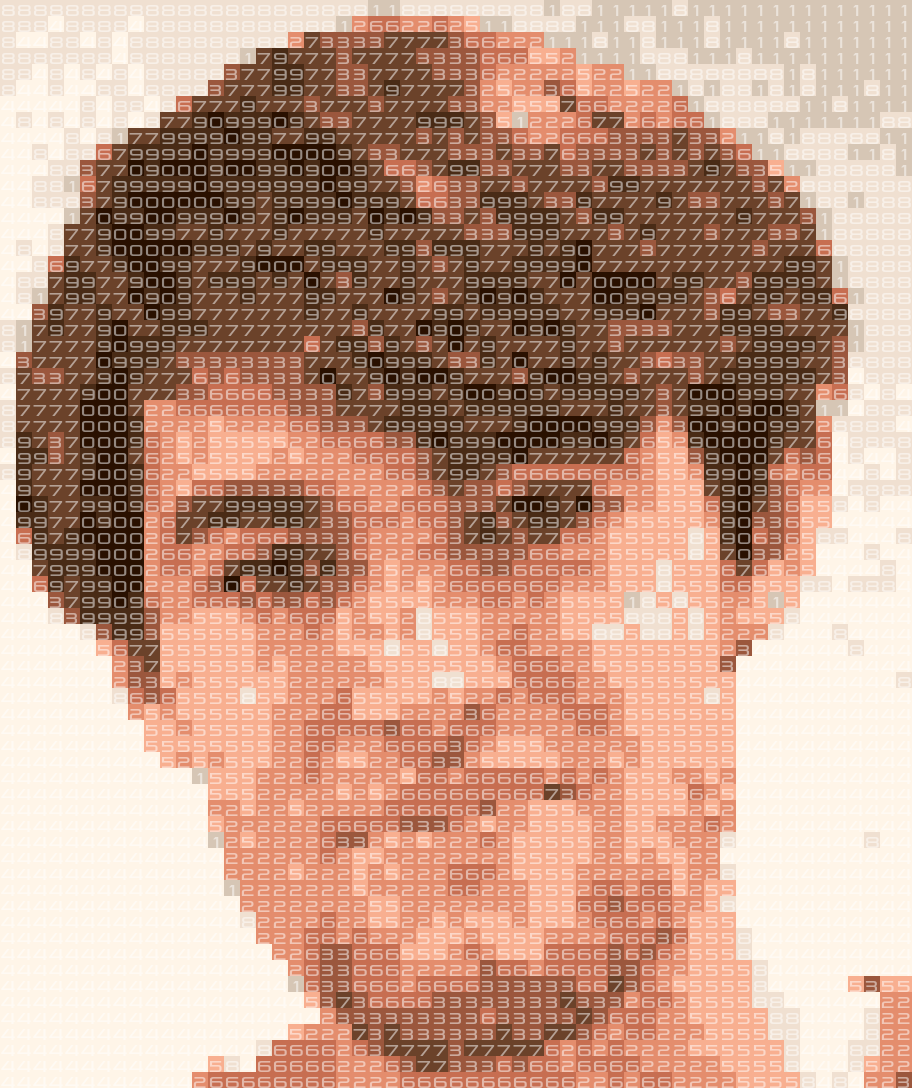## Requests

As I put the code online you can try to generate some prime portraits for yourself! If you really think I should try a certain artwork, feel free to reach out to me. Also: if you have a good idea what to do with these results, feel free to reach out. I know that I personally am going to hang the prime version of Starry Night on my wall!

## 2 comments for “Painting by Prime Number”

1.June 8, 2018 at 5:14 pm

A couple of thoughts:

* have you tried clustering in a different colorspace than RGB to see if it looks better?
* instead of regenerating the entire image over and over, pick an image you like, and then try relabeling the 10 digits to search for primes. Since the last digit cannot be even or 5, there are only 4 * 9 * 8 * 7 * 6 * 5 * 4 * 3 * 2 = 1451520 relabelings that could be prime. That might be faster to check.

2.Robert G.
April 18, 2020 at 12:11 am

If you want numbers that are provably prime, you can stick a buffer that’s half the height of the image on the bottom and use the Pocklington primality test. Essentially, if a third of the digits of the number are able to be factored out if you add or subtract one from the number, you can prove conclusively whether the number is prime or not. For an image like this, if you make the bottom third of the image all 9s or all 0s with a 1 at the end, you can use the Pocklington test (implemented in pfgw as -t / -tm). I think this is slightly cooler, since in the hobby prime crunching world, proved primes tend to be more valuable than PRPS (although there are plenty of primality projects that use PRPs, don’t get me wrong)